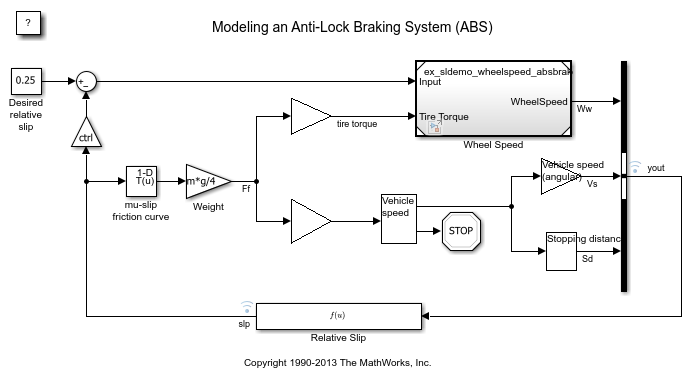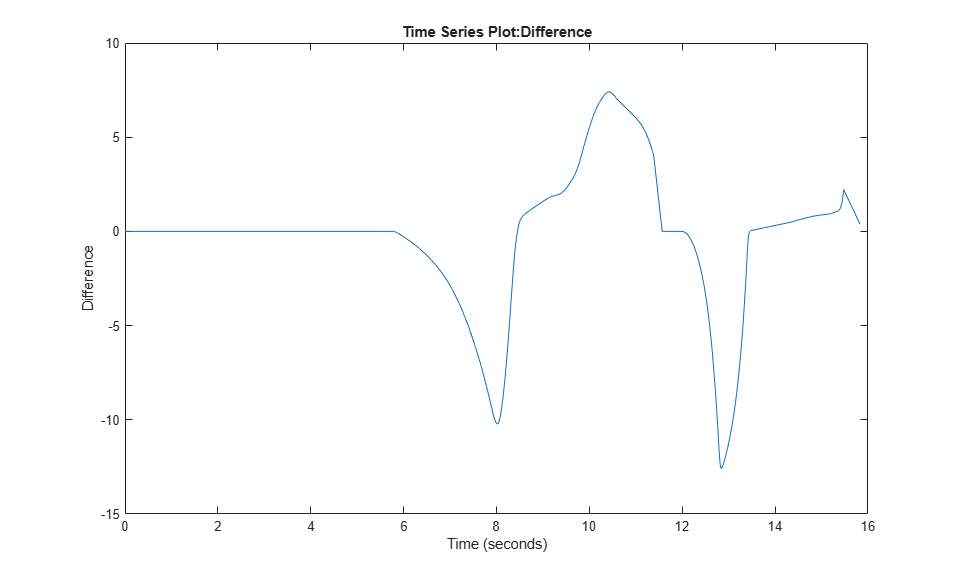## Optimize, Estimate, and Sweep Block Parameter Values

When you sweep one or more parameters, you change their values between simulation runs, and compare and analyze the output signal data from each run. Use parameter sweeping to tune control parameters, estimate unknown model parameters, and test the robustness of a control algorithm by taking into consideration uncertainty in the real-world system.

You can sweep block parameter values or the values of workspace variables that you use to set the parameter values. Use the Parameters tab on the Model Data Editor (on the Modeling tab, click Model Data Editor), the Property Inspector (on the Modeling tab, under Design, click Property Inspector), the command prompt, or scripts to change parameter values between simulation runs.

If you want to repeatedly change the value of a block parameter, consider creating a variable in a workspace. You can use the Model Explorer or programmatic commands to change the value of the variable instead of locating or identifying the block in the model. Also, several features and products that facilitate parameter optimization, estimation, and sweeping require that you set block parameter values by creating workspace variables.

To learn how to manipulate parameter values during the iterative process of creating a model, see Tune and Experiment with Block Parameter Values.

For basic information about accessing and setting block parameter values as you design a model, see Set Block Parameter Values. For basic information about programmatically simulating a model, such as by using a script, see Run Simulations Programmatically.

### Sweep Parameter Value and Inspect Simulation Results

This example shows how to change a block parameter value between multiple programmatic simulation runs. Use this technique to determine an optimal parameter value by comparing the output signal data of each run.

The example model `ex_sldemo_absbrake` uses a Constant block to specify a slip setpoint for an anti-lock braking system. Simulate the model with two different slip setpoint values, 0.24 and 0.25, and compare the output wheel speed of each simulation run.

To store the setpoint value, create a variable in the base workspace. This technique enables you to assign a meaningful name to the value.

Open the example model.

```open_system('ex_sldemo_absbrake'); ```On the Modeling tab, click Model Data Editor.

In the Model Data Editor, select the Signals tab.

Set the Change view drop-down list to `Instrumentation`. The Log Data column shows that the signals `yout` (which is a virtual bus) and `slp` are configured for logging. When you simulate the model, you can collect and later inspect the values of these signals by using the Simulation Data Inspector.

In the Model Data Editor, select the Parameters tab. Set Change view to `Design`.

In the model, select the Constant block labeled `Desired relative slip`. The Model Data Editor highlights the row that corresponds to the Constant value parameter of the block.

Use the Value column to set the parameter value to `relSlip`.

While editing the value, next to `relSlip`, click the action button (with three vertical dots) and select Create.

In the Create New Data dialog box, set Value to `0.2` and click Create. A variable, whose value is `0.2`, appears in the base workspace. The model now acquires the relative slip setpoint from this variable.

Alternatively, you can use these commands at the command prompt to create the variable and configure the block:

```relSlip = 0.2; set_param('ex_sldemo_absbrake/Desired relative slip','Value','relSlip') ```

At the command prompt, create an array to store the two experimentation values for the relative slip setpoint, `0.24` and `0.25`.

```relSlip_vals = [0.24 0.25]; ```

Create a `Simulink.SimulationInput` object for each simulation that you want to run (in this case, two). Store the objects in a single array variable, `simIn`. Use the `setVariable` method of each object to identify each of the two experimentation values.

```for i = 1:length(relSlip_vals) simIn(i) = Simulink.SimulationInput('ex_sldemo_absbrake'); simIn(i) = setVariable(simIn(i),'relSlip',relSlip_vals(i)); end ```

Use the `sim` function to simulate the model. Optionally, store the output in a variable named `simOutputs`.

```simOutputs = sim(simIn); ```
```[23-Feb-2021 19:05:32] Running simulations... [23-Feb-2021 19:05:35] Completed 1 of 2 simulation runs [23-Feb-2021 19:05:36] Completed 2 of 2 simulation runs ```

The model streams the logged signals, `yout` and `slp`, to the Simulation Data Inspector. You can view the signal data in the Simulation Data Inspector.

Compare the output data of the two latest simulation runs.

```runIDs = Simulink.sdi.getAllRunIDs(); runResult = Simulink.sdi.compareRuns(runIDs(end-1), runIDs(end)); ```

Plot the difference between the values of the `Ww` signal (which is an element of the virtual bus signal `yout`) by specifying the result index `1`.

```signalResult = getResultByIndex(runResult,1); plot(signalResult.Diff); ```### Store Sweep Values in `Simulink.SimulationInput` Objects

When you write a script to run many simulations, create an array of `Simulink.SimulationInput` objects (one object for each simulation that you want to run). Use the `setVariable` and `setBlockParameter` methods of each object to identify the parameter values to use for the corresponding simulation run. With this technique, you avoid having to use the `set_param` function to modify block parameter values and assignment commands to modify workspace variable values between simulation runs.

For more information about using `Simulink.SimulationInput` objects to run multiple simulations, see `sim`.

#### Sweep Nonscalars, Structures, and Parameter Objects

If you use nonscalar variables, structure variables, or `Simulink.Parameter` objects to set block parameter values, use the `setVariable` method of each `Simulink.SimulationInput` object. Refer to the examples in the table.

ScenarioExample
MATLAB® variable, `myArray`, whose value is an array. You want to set the third element in the array (assuming one-based indexing).
`setVariable(simIn,'myArray(3)',15.23)`
MATLAB variable, `myStruct`, that has a field named `field1`.
`setVariable(simIn,'myStruct.field1',15.23)`
Parameter object, `myParam`, whose `Value` property is a scalar.
`setVariable(simIn,'myParam.Value',15.23)`
Parameter object, `myArrayParam`, whose `Value` property is an array. You want to set the third element in the array.
`setVariable(simIn,'myArrayParam.Value(3)',15.23)`
Parameter object, `myStructParam`, whose `Value` property is a structure. The structure has a field named `field1`.
`setVariable(simIn,'myStructParam.Value.field1',15.23)`

#### Sweep Value of Variable in Model Workspace

If you use the model workspace to store variables, when you use the `setVariable` method of a `Simulink.SimulationInput` object to modify the variable value, use the `Workspace` pair argument to identify the containing model:

`setVariable(simIn,'myVar',15.23,'Workspace','myModel')`

### Capture and Visualize Simulation Results

Each simulation run during a parameter sweep produces outputs, such as signal values from Outport blocks and from logged signals.

You can capture these outputs in variables and objects for later analysis. For more information, see Export Simulation Data.

To visualize simulation output data so you can compare the effect of each parameter value, see Decide How to Visualize Simulation Data.

### Improve Simulation Speed

To perform many simulations that each use different parameter values, you can use accelerated simulation modes. For larger models, accelerated simulations take less time to execute than normal simulations. If you also have Parallel Computing Toolbox™, you can use the multiple cores of your processor to simultaneously execute simulations. Use arguments of the `sim` and `parsim` functions.

To improve the simulation speed of your model by using accelerated simulations and other techniques, see Optimize Performance. For examples and more information, see Run Multiple Simulations.

### Sweep Parameter Values to Test and Verify System

If you have Simulink® Test™, you can confirm that your model still meets requirements when you use different parameter values. Parameter overrides and test iterations enable you to set different parameter values for each test case. For more information, see Parameter Overrides (Simulink Test) and Test Iterations (Simulink Test).

### Estimate and Calibrate Model Parameters

If you have Simulink Design Optimization™, you can estimate model parameter values so that simulation outputs closely fit the data that you measure in the real world. Use this technique to estimate the real-world values of parameters in a plant model, which represents the dynamics of a real-world system, when you cannot directly measure the values. This estimation improves the accuracy of the plant model. For more information, see Estimate Parameters from Measured Data (Simulink Design Optimization).

### Tune and Optimize PID and Controller Parameters

If you have Simulink Control Design™, you can use PID Tuner to tune the parameters of a PID Controller block. For more information, see PID Controller Tuning in Simulink (Simulink Control Design).

If you have Simulink Design Optimization, you can optimize control parameter values so that simulation outputs meet response requirements that you specify. For more information, see Design Optimization to Meet Step Response Requirements (GUI) (Simulink Design Optimization).